# Functional programming in JavaScript

Functions are the most important part of functional programming (especially in JavaScript). Functions are the single source that helps developers to perform functional programming. Generally abbreviated as FP which revolves around functions and it is how we use functions that makes our code functional. Without function, there is no FP. So the question comes what exactly is a function?

In simple terms, a function is a collection of code that can be executed several times. Most of us confuse a function with a procedure. But they are separate entities.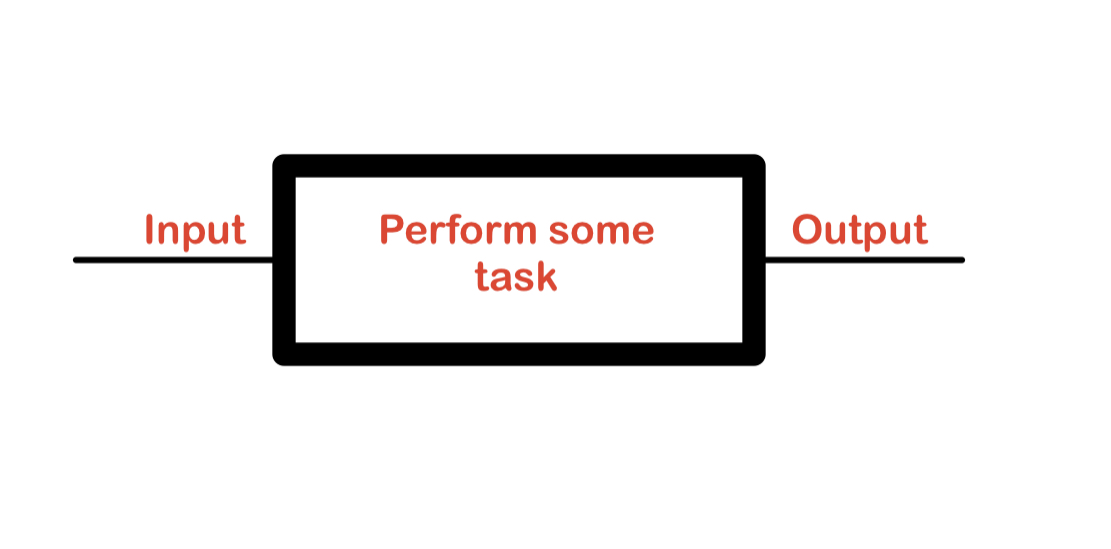The high-level view of a function.

In functional programming, we should use functions as much as possible.

Input: In general programming, we interchangeably use arguments with parameters but they are not the same ones. Arguments are data that we pass in via function call and parameters are data we receive in a function definition.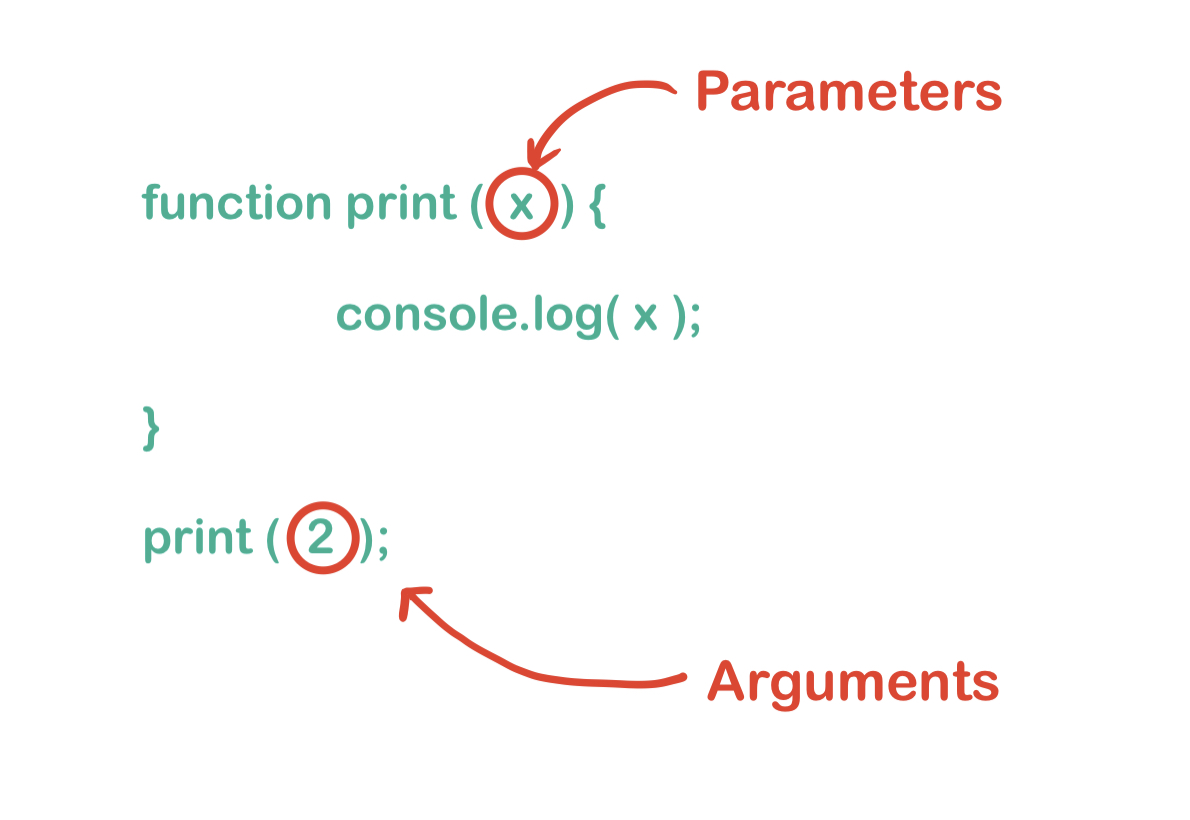Difference between Parameters and Arguments

In JavaScript, it is not necessary that the number of arguments are always the same as parameters. If we pass fewer arguments then the remaining parameters will get undefined as a value in function and if we pass more arguments than declared parameters then the remaining arguments remain untouched.

We can get all arguments without even defining a single parameter, using arguments as array-like object, but it is not a good practice.

Do you know every function has an internal property named arguments. Have you ever notice why it is called arguments not parameters?

Note: An Argument is an array-like object that we can access inside a function. It contains properties that are passed to a function. Since we are talking about the properties that are passed to function not the properties that receive properties as parameters.

1. An argument is an array-like object. We can access values with an array index but cannot use array methods like forEach, map, reduce, etc. as it is array-like but not array.
2. An argument object contains values of the arguments passed to that function (So it is called arguments). In the below program, we can see the output of arguments. It prints all values that we pass as arguments.

## Javascript

 `function` `print(a, b) { ` `  ``console.log(a);  ` `  ``console.log(b);  ` ` `  `  ``console.log(arguments);      ` ` `  `  ``console.log(arguments);  ` `  ``console.log(arguments);  ` `} ` ` `  `print(1, 2, 3, 4); `

Output: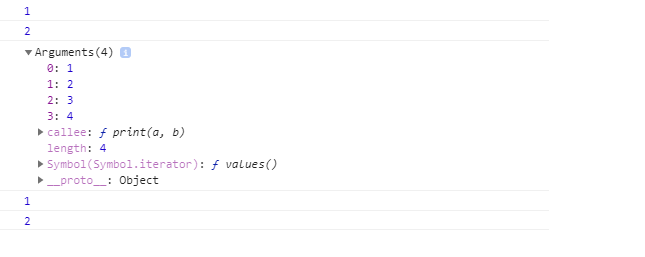Note: As of ES5, using arguments is not considered a good practice. You should avoid using it. But if you use arguments, the object is limited to length property.

In functional programming, we strictly take care of the number of parameters in a function declaration that is known as arity. We have to take care of arity because sometimes we have to pass a function inside a function then we have to think in terms of compatibility.

## Javascript

 `// The arity of maipulateJSON is 2. we can find ` `// arity with length property of a function ` `function` `manipulateJSON(json, options) { ` `    ``console.log(json, options) ` `} ` ` `  `console.log( manipulateJSON.length )`

Output:

`2`

Function with arity 1 is known as unary function, Similarly function with arity 2 is known as binary, and with more that 2, the arity is known as n-ary function.

There could be a problem with the length function. Let’s say, if there is a default parameter or rest parameter then that parameter does not include in the length.

## Javascript

 `function` `printDefault(fn, args = {}) { ` `  ``fn(args); ` `} ` ` `  `function` `printRest(fn, ...args) { ` `  ``fn(...args); ` `} ` ` `  `printDefault(console.log, { name: ``"Praveen"` `}); ` `printRest(console.log, 1, 2, 3, 4); ` ` `  `console.log(printDefault.length);  ` `console.log(printRest.length);        `

Output: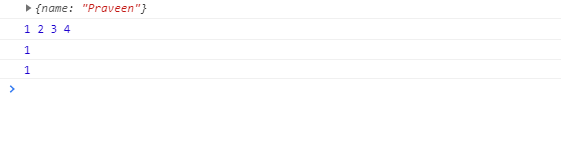Note: In JavaScript, function always returns output either externally(by JS engine) or internally (by developer).

## Javascript

 `function` `print1( name ) { ` `  ``console.log( name ); ` `} ` ` `  `function` `print2( name ) { ` `  ``console.log( name ); ` `  ``return``; ` `} ` ` `  `function` `print3( name ) { ` `  ``console.log( name ); ` `  ``return` `undefined; ` `} ` `console.log( print1(``"Praveen"``) ); ` `console.log( print2(``"Praveen"``) ); ` `console.log( print3(``"Praveen"``) );`

Output:Note: It is recommended to return a value from a function in functional programming. In a case you want to return multiple values then you could use an array or an object.

You know what in JavaScript, the return keyword not only uses a function to return values but can also be used in the flow control statement. That means we can just stop the execution of a function at any place or anywhere in a function execution. There could be a case where there may be multiple return statements in a function. But it just confuses the developer to figure out how the function works. It’s just, not a good practice to use multiple returns in a function.

Function can return value(s) As we all know function can return value(s). It can be a primitive, array or it could be an object.

Note: Do you prefer semicolon(;) while writing JavaScript. If YES then it is great, but if in case NO then my friend you should. I know it should be a developer preference, but in JavaScript it is recommended to use semicolons. JavaScript uses Automatic semicolon insertion(ASI). If you’re not inserting a semicolon then ASI comes into action and there could be a repercussion. There could be some serious bugs.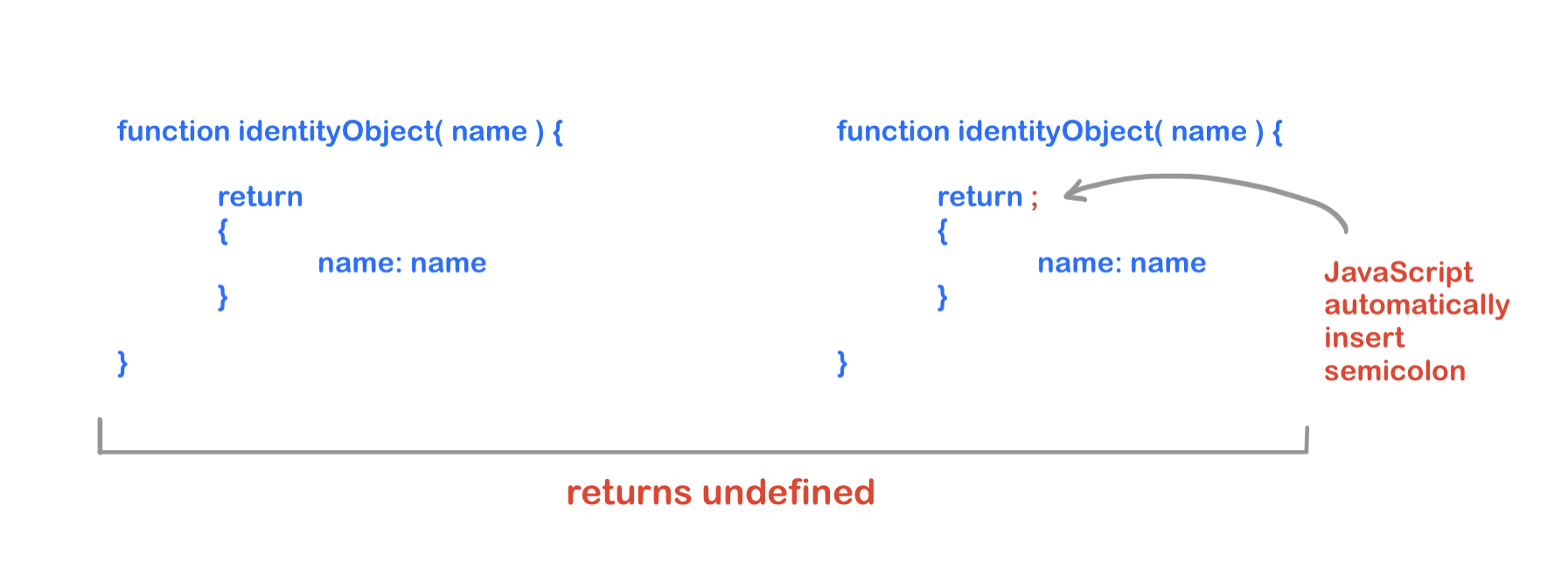function after Automatic semicolon Insertion(ASI)

You must be expecting identityObject returns an object but instead, it will return undefined.

JavaScript engine is smart enough and know where to insert semicolon. But why to depend on engine.

#### A function can return another function

If a function that returns or receives one or more function values, then that function is known as a higher-order function

## Javascript

 `const arr = [1, 2, 3, 4, 5, 6]; ` ` `  `arr.forEach(``function` `printArrayValues(val) { ` `  ``console.log(val); ` `});`

Output:In the above program, arr is an array, and we are using the forEach method to print all values. The forEach() is a higher-order function and expects a function as parameters.

We are using higher-order functions in day to day life and FP is no exceptional. FP uses higher-order in every place. Now it is time to introduce closure.

Closure is all about remembering the scope variables even after the scope has changed. We cannot do FP without closure and closure is all about returning a function from another function and remember the scope. We will discuss closure in another article.

ES6 Arrow function: We all have heard about the arrow function. As it has shorter syntax and no function keyword.

## Javascript

 `const numbers = [1, 2, 3, 4, 5, 6]; ` ` `  `const newNumbers = numbers.map(``function` `(x) { ` `  ``return` `x * 2; ` `}); ` `console.log(newNumbers);     ` ` `  `const arrowNewNumbers = numbers.map((x) => x * 2); ` `console.log(arrowNewNumbers);     `

Output: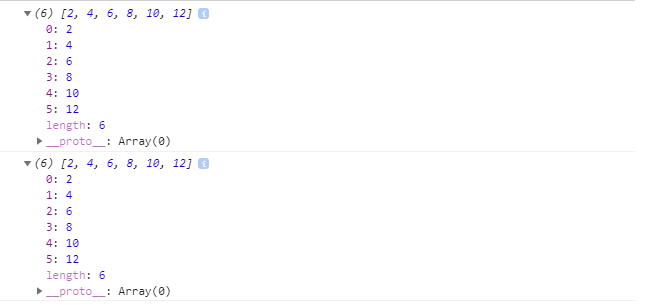Most of the user prefers the arrow function, but I do not recommend using it. Below are the reasons

• Debugging is not easy with the arrow function, because the arrow function is an anonymous function and if in any case we’ll get some bug, and we debug our application then in function stack we won’t get the function name.
• We always have to take care of the syntax of the arrow function described below.

Rules of arrow function:

• We can drop the function keyword.
• We can drop the return keyword if there is a single statement in the arrow function.
• We have to use the return keyword if we want to return an object.

## Javascript

 `const numbers = [1, 2, 3, 4, 5, 6]; ` ` `  `// We can drop function and return keyword ` `const version1 = numbers.map((x) => x * 2); ` ` `  `// If there is single parameter thern we  ` `// can also drop parenthesis ` `const version2 = numbers.map(x => x * 2); ` ` `  `// If we have to return an object then we ` `// have to use curly braces with return  ` `// keyword because compiler gets easily ` `// confused with a block. ` `// const version3 = (arr) => { array: arr } // ERROR ` `const version3 = ``function` `(arr) { ` `  ``return` `{ ` `    ``array: arr, ` `  ``}; ` `}; ` ` `  `console.log(version1); ` `console.log(version2); ` ` `  `console.log(version3(numbers)); `